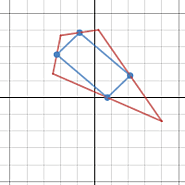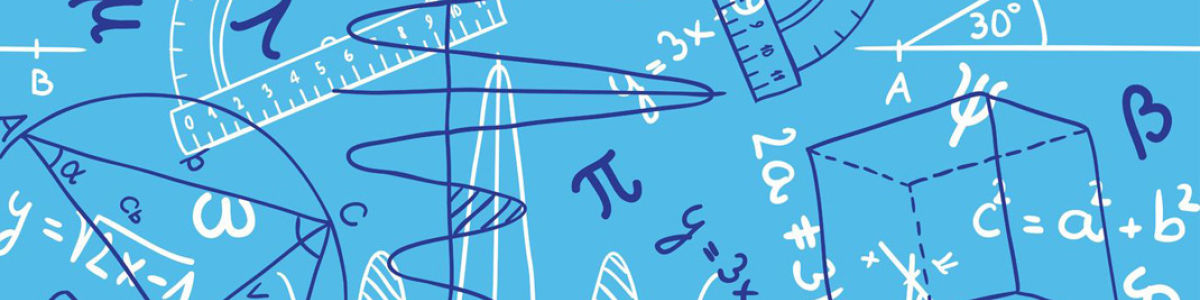Updated by Desmos Links on Apr 15, 2019
REPORT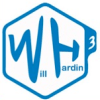Desmos Links
Owner
20 items   1 followers   0 votes   10 views

Geometry

1

Altitudes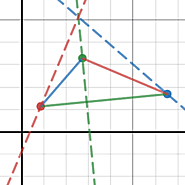Altitudes

2

Perpandicular Bisectors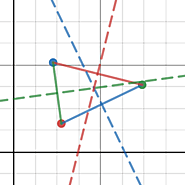Perpandicular Bisectors

3

Medians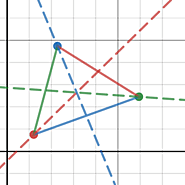Medians

4

Locus: Point and Line Segment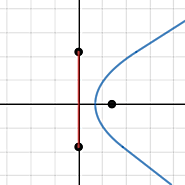Locus: Point and Line Segment

5

Area of a Triangle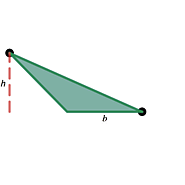Area of a Triangle

6

Equation of a circle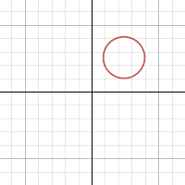Equation of a circle

8

What's My Transformation? • Activity Builder by Desmos9

Polygraph by Desmos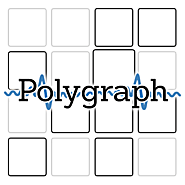With Polygraph, Desmos provides tools for developing informal language into formal vocabulary. Because words should result from a need to describe our world—this is where they gain their power.

10

Angle Relationships • Polygraph by Desmos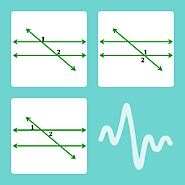11

Geometry Venn Diagram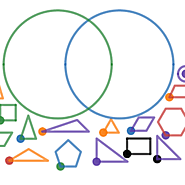Geometry Venn Diagram

12

Shape Bucket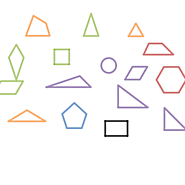Shape Bucket

13

Exterior Angles: Triangles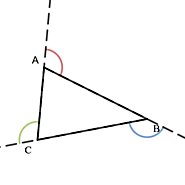Exterior Angles: Triangles

14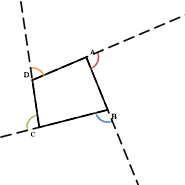15

Exterior Angles: Pentagons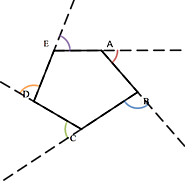Exterior Angles: Pentagons

16

Exterior Angles: Hexagons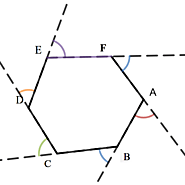Exterior Angles: Hexagons

17

Triangle Angle Sum Theorem eTool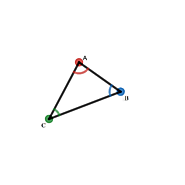Triangle Angle Sum Theorem eTool

18

Area of a Triangle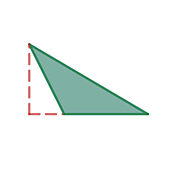Area of a Triangle

19

Triangle Inequality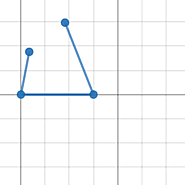Triangle Inequality

20

Pythagorean Theorem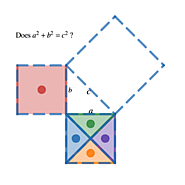Pythagorean Theorem

21